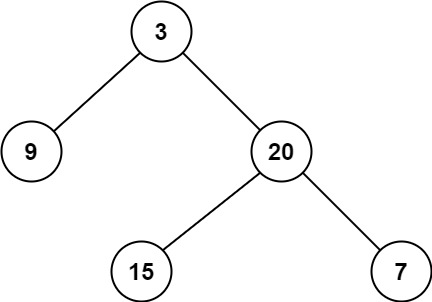# 111.minimum-depth-of-binary-tree

## Statement

• Difficulty: Easy
• Tag: `树` `深度优先搜索` `广度优先搜索` `二叉树```````输入：root = [3,9,20,null,null,15,7]

``````

``````输入：root = [2,null,3,null,4,null,5,null,6]

``````

• 树中节点数的范围在 `[0, 105]`
• `-1000 <= Node.val <= 1000`

• Link: Minimum Depth of Binary Tree
• Difficulty: Easy
• Tag: `Tree` `Depth-First Search` `Breadth-First Search` `Binary Tree`

Given a binary tree, find its minimum depth.

The minimum depth is the number of nodes along the shortest path from the root node down to the nearest leaf node.

Note: A leaf is a node with no children.

Example 1:``````Input: root = [3,9,20,null,null,15,7]
Output: 2
``````

Example 2:

``````Input: root = [2,null,3,null,4,null,5,null,6]
Output: 5
``````

Constraints:

• The number of nodes in the tree is in the range `[0, 105]`.
• `-1000 <= Node.val <= 1000`

## Solution

``````# Definition for a binary tree node.
# class TreeNode:
#     def __init__(self, val=0, left=None, right=None):
#         self.val = val
#         self.left = left
#         self.right = right
class Solution:
def __init__(self):
self.res = 1000000

def dfs(self, rt: TreeNode, d: int) -> None:
if not rt.left and not rt.right:
self.res = min(self.res, d)

if rt.left:
self.dfs(rt.left, d + 1)

if rt.right:
self.dfs(rt.right, d + 1)

def minDepth(self, root: TreeNode) -> int:
if not root:
return 0

self.dfs(root, 1)
return self.res
``````